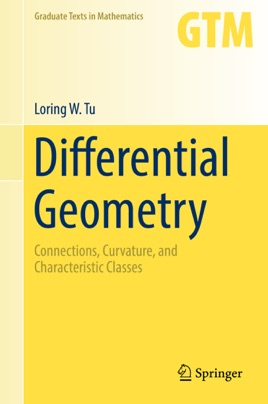• \$44.99

Publisher Description

This text presents a graduate-level introduction to differential geometry for mathematics and physics students. The exposition follows the historical development of the concepts of connection and curvature with the goal of explaining the Chern–Weil theory of characteristic classes on a principal bundle. Along the way we encounter some of the high points in the history of differential geometry, for example, Gauss' Theorema Egregium and the Gauss–Bonnet theorem. Exercises throughout the book test the reader’s understanding of the material and sometimes illustrate extensions of the theory. Initially, the prerequisites for the reader include a passing familiarity with manifolds. After the first chapter, it becomes necessary to understand and manipulate differential forms. A knowledge of  de Rham cohomology is required for the last third of the text.

Prerequisite material is contained in author's text An Introduction to Manifolds, and can be learned in one semester. For the benefit of the reader and to establish common notations, Appendix A recalls the basics of manifold theory. Additionally, in an attempt to make the exposition more self-contained, sections on algebraic constructions such as the tensor product and the exterior power are included.

Differential geometry, as its name implies, is the study of geometry using differential calculus. It dates back to Newton and Leibniz in the seventeenth century, but it was not until the nineteenth century, with the work of Gauss on surfaces and Riemann on the curvature tensor, that differential geometry flourished and its modern foundation was laid. Over the past one hundred years, differential geometry has proven indispensable to an understanding of the physical world, in Einstein's general theory of relativity, in the theory of gravitation, in gauge theory, and now in string theory. Differential geometry is also useful in topology, several complex variables, algebraic geometry, complex manifolds, and dynamical systems, among other fields.  The field has even found applications to group theory as in Gromov's work and to probability theory as in Diaconis's work. It is not too far-fetched to argue that differential geometry should be in every mathematician's arsenal.